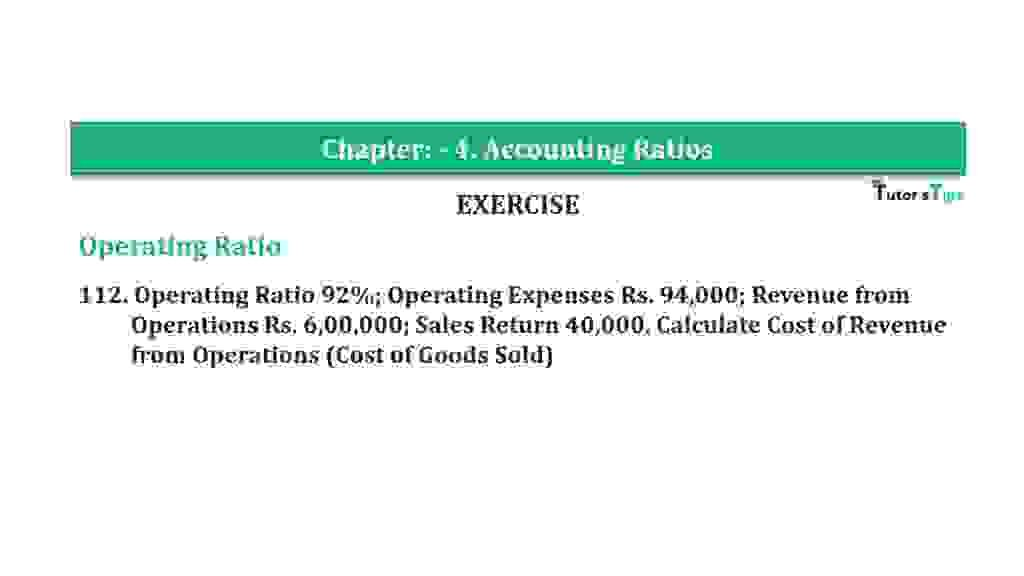# Question 112 Chapter 4 of +2-B – T.S. Grewal 12 ClassQuestion 112 Chapter 4 of +2-B

Operating Ratio

112. Operating Ratio 92%; Operating Expenses Rs. 94,000; Revenue from
Operations Rs. 6,00,000; Sales Return 40,000. Calculate Cost of Revenue
from Operations (Cost of Goods Sold)

### The solution of Question 112 Chapter 4 of +2-B: –

 Net Sales = Rs. 6,00,000 Operating Ratio = 92%

 Revenue from Operations = Gross Profit X 100 Net Sales
 92% = Operating Cost X 100 Rs. 5,00,000 = 84%

 Operating Cost = Rs. 6,00,000 x 92% Operating Cost = Rs. 5,52,000 Operating Cost = Cost of Goods Sold + Operating Expenses Rs. 5,52,000 = Cost of Goods Sold + Rs. 94,000 Cost of Goods Sold = Rs. 5,52,000 – Rs. 94,000 = Rs. 4,58,000

Balance Sheet: Meaning, Format & Examples

Thanks, Please Like and share with your friends

Comment if you have any question.

Also, Check out the solved question of previous Chapters: –

### T.S. Grewal’s Double Entry Book Keeping (Vol. II: Accounting for Companies)T.S. Grewal’s Analysis of Financial Statements